# GED Math : Representing Data

## Example Questions

### Example Question #1 : Bar Graphs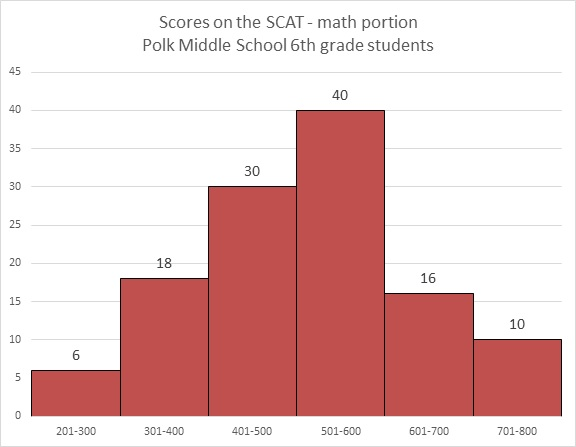Refer to the above graph. Yasmin, a sixth grader at Polk, outscored 89 of the students who took the test. Which of these could her score have been?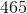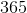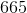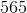Explanation: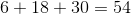students achieved scores between 200 and 500, and Yasmin outscored all of them.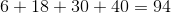students achieved scores between 200 and 600, but Yasmin did not outscore all of them.

Her score fell in the range between 501 and 600, so of the four choices, the only plausible score was 565.

### Example Question #1 : Bar GraphsWhich question cannot be answered about Polk Middle School sixth graders' performance on the math portion of the SCAT by looking at the above bar graph?

How many students scored above 300 on the test?

What fraction of the students scored at 600 or on the test?

What percent of the students scored from 401 to 600 on the test?

What was the lowest score on the test?

What was the lowest score on the test?

Explanation:

The lowest score is not indicated anywhere on the graph, so the question "What was the lowest score on the test?" is the one that cannot be answered. The other three can be determined, in part or completely, by adding the number of students in one or more ranges, and in two cases, also adding the number of students total.

### Example Question #3 : Bar GraphsWhich question can be answered about Polk Middle School sixth graders' performance on the math portion of the SCAT by looking at the above bar graph?

How many students took the test?

What was the mode of the scores on the test?

What was the median score on the test?

What was the mean score on the test?

How many students took the test?

Explanation:

The graph only provides information about how many students scored in each range (201-300, 301-400, etc.) on the test; it provides no information about the mean, median, or mode. The only question that can be answered is the number of students total who took the test - this can be answered by adding the numbers on top of the bars.

### Example Question #11 : Bar Graphs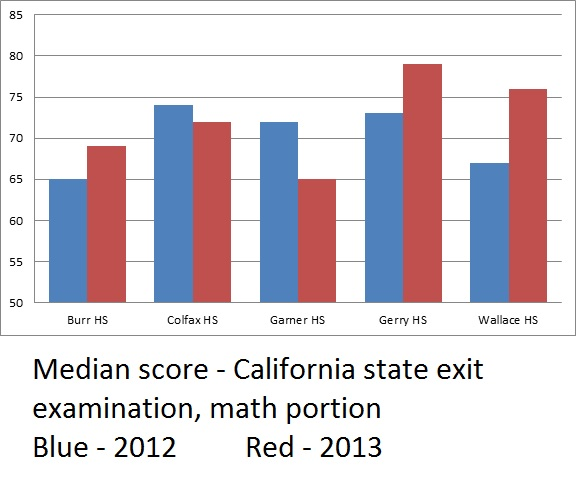The above bar graph compares exit examination scores from both 2012 and 2013 for the five high schools in a school district.

Which school saw the most improvement in its median math score between 2012 and 2013?

Burr

Gerry

None of the schools saw improvement

Wallace

Wallace

Explanation:

Wallace, Gerry, and Burr all saw improvement, as the bars representing their 2013 median scores are longer than the ones for 2012. A visual inspection shows that the greatest difference is between the two bars representing Wallace High, so Wallace is the correct choice.

### Example Question #2081 : Ged Math

Use the following bar graph to answer the question: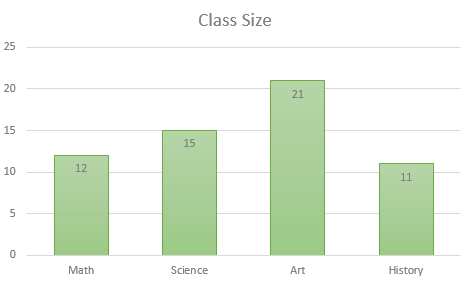How many students are in Art class?Explanation:

Let's look at the bar graph.The bottom of the bar graph lists the different classes. The height of each bar displays a quantity that matches up with the scale on the left side. This quantity represents the number of students in that class.

You can either use the left scale to determine the number of students, or you can see the quantities posted on each bar.

So, we will find the bar that corresponds to Art class. We can see it is the 3rd class listed. Now, we can see that bar goes up to 21.

Therefore, the number of students in Art class is 21.

### Example Question #11 : Bar Graphs

Use the following bar graph to answer the question: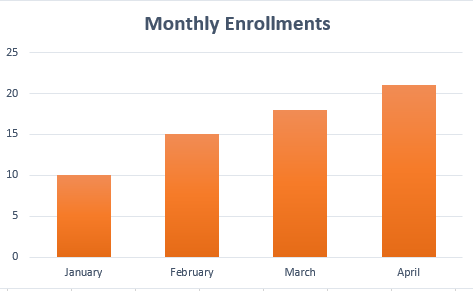Approximately, how many enrollments were there in April?Explanation:

Let's look at the bar graph.We can see the months are listed along the bottom axis. We can also see the scale on the left ranges from 0 to 25. These numbers represent the number of enrollments.

Now, to find the approximate number of enrollments for April, we will first find April. We can see it is the last month listed.

Now, we can see the value of the bar is between 20 and 25. We can also see that it is fairly close to 20.

Therefore, we can approximate the number of enrollments for April is 21.

### Example Question #12 : Bar Graphs

Use the following bar graph to answer the question: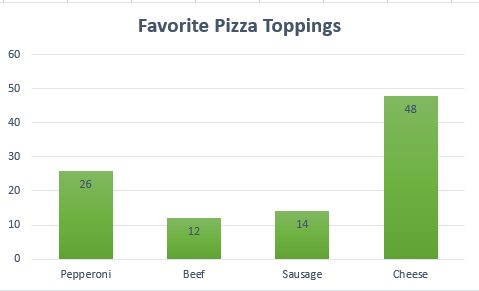How many more people like cheese than pepperoni?Explanation:

Let's look at the bar graph.We can see the different pizza toppings on listed on the bottom. We can also see the number of people who like a specific pizza topping is shown on the bar.

Now, we want to find how many more people like cheese than pepperoni. To d that, we need to find the difference of those who like cheese and those who like pepperoni.

So, we can see cheese is the last topping listed. It shows 48 people. We can see pepperoni is the first topping listed. It shows 26 people like it.

Now, we will find the difference. We get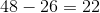Therefore, 22 more people like cheese than pepperoni.

### Example Question #32 : Representing Data

Use the following bar graph to answer the question: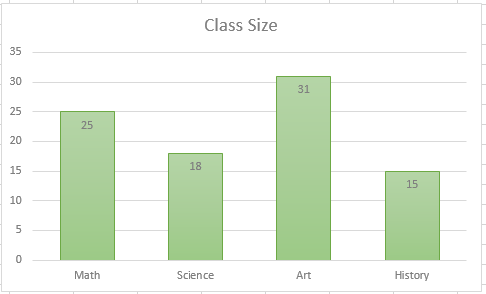How many students are in the largest class?Explanation:

Let's look at the bar graphTo find the number of students in the largest class, we will essentially be looking at the class with the most students (or the class with the tallest bar).

We can see the class with the tallest bar is Art. We can see it has 31 students.

Therefore, the largest class has 31 students.

### Example Question #12 : Bar Graphs

Use the following bar graph to answer the question: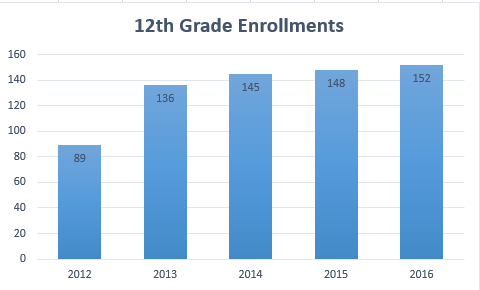What is the difference of 12th grade enrollments from the year 2012 to the year 2015?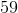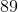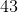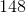Explanation:

Let's look at the graph:We can see the bars represent the number of enrollments in the 12th grade for different years.

To find the difference of enrollments from 2012 to 2015, we will first find the number of enrollments from those years, then we will subtract them.

So, we can see the number of enrollments in 2012 was 89. We can see the number of enrollments in 2015 was 148. Now, we subtract. We get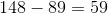Therefore, the difference of 12th grade enrollments from 2012 to 2015 is 59.

### Example Question #13 : Bar Graphs

Use the following bar graph to answer the question:Which year had the most enrollments?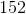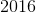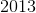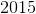Explanation:

Let's look at the graph.To find the year with the most enrollments, we will find the year that has the tallest bar.

We can see the tallest bar shows 152 enrollments. It corresponds to the year 2016.

Therefore, the year with the most enrollments is 2016.

### All GED Math Resources